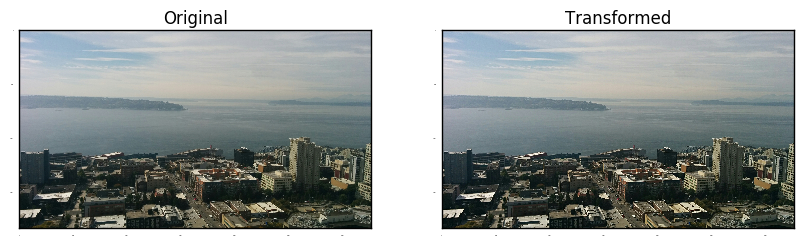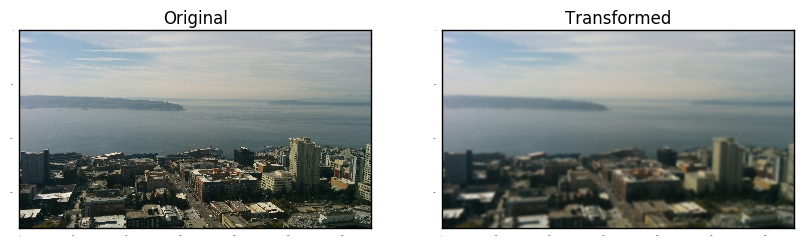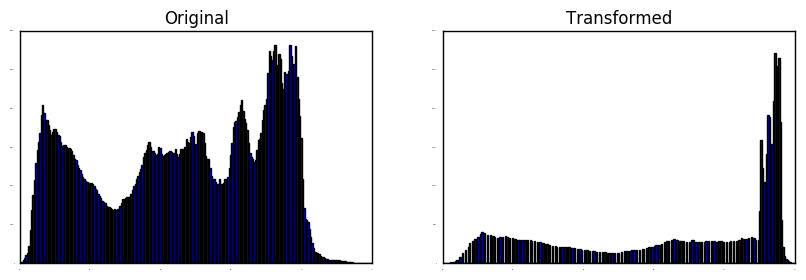# Instagram Filters in Python¶

Michele Pratusevich

• By day: computer vision researcher at Amazon
• By night: maintainer of Practice Python blog

Reach me:

• mprat@alum.mit.edu
• @practice_python

The basic ingredients of any Instagram filter:

• Sharpening / blurring
• Adjusting individual channels by linear interpolation

Let's set up our variables and load our image.

In :
import matplotlib
matplotlib.use('Agg')
%matplotlib inline
import matplotlib.pyplot as plt
import skimage
from skimage import io
from skimage import filters
import numpy as np

In :
matplotlib.rcParams['xtick.major.size'] = 0
matplotlib.rcParams['ytick.major.size'] = 0
matplotlib.rcParams['xtick.labelsize'] = 0
matplotlib.rcParams['ytick.labelsize'] = 0

In :
def plot_side_by_side(first, second, t):
if t == 'image':
f, (ax1, ax2) = plt.subplots(1, 2, figsize=(10, 5))
ax1.imshow(first)
ax2.imshow(second)
elif t == 'hist':
f, (ax1, ax2) = plt.subplots(1, 2, figsize=(10, 3))
(_, _, _) = ax1.hist(first.flatten(), bins=255)
(_, _, _) = ax2.hist(second.flatten(), bins=255)
ax1.set_title("Original")
ax2.set_title("Transformed")
plt.show()


Sharpening: Take a blurred version of an image and subtract it from the original image. Can scale the blurred / original image as needed.

In :
def sharpen(image, a, b, sigma=10):
blurred = skimage.filters.gaussian(image, sigma=sigma, multichannel=True)
sharper = np.clip(image * a - blurred * b, 0, 1.0)
return sharper

In :
sharpened = sharpen(original_image, 1.3, 0.3)
plot_side_by_side(original_image, sharpened, "image")(Yes, you can also use this same function to just blur your image if you want to)

In :
blurred = sharpen(original_image, 0, -1.0)
plot_side_by_side(original_image, blurred, "image")## Adjusting channels by linear interpolation¶

The range [0, 1] is broken into the range of buckets and linearly interpolate between them. It's like the same as a curve adjustment in any photo editing software.

In :
plot_side_by_side(r, r2, "hist")
plt.show()Let's make a function that takes a single channel of an image and adjust it according to a list of values.

In :
def channel_adjust(channel, values):
# flatten
orig_size = channel.shape
flat_channel = channel.flatten()
flat_channel,
np.linspace(0, 1, len(values)),
values)

# put back into image form


One more set of helper functions.

In :
# skimage loads images in RGB format
def split_image_into_channels(image):
red_channel = image[:, :, 0]
green_channel = image[:, :, 1]
blue_channel = image[:, :, 2]
return red_channel, green_channel, blue_channel

In :
# and we'll have to undo it
def merge_channels(red_channel, green_channel, blue_channel):
return np.stack([red_channel, green_channel, blue_channel], axis=2)


So you believe this works:

In :
r, g, b = split_image_into_channels(original_image)
im = merge_channels(r, g, b)
plt.imshow(im)
plt.show()